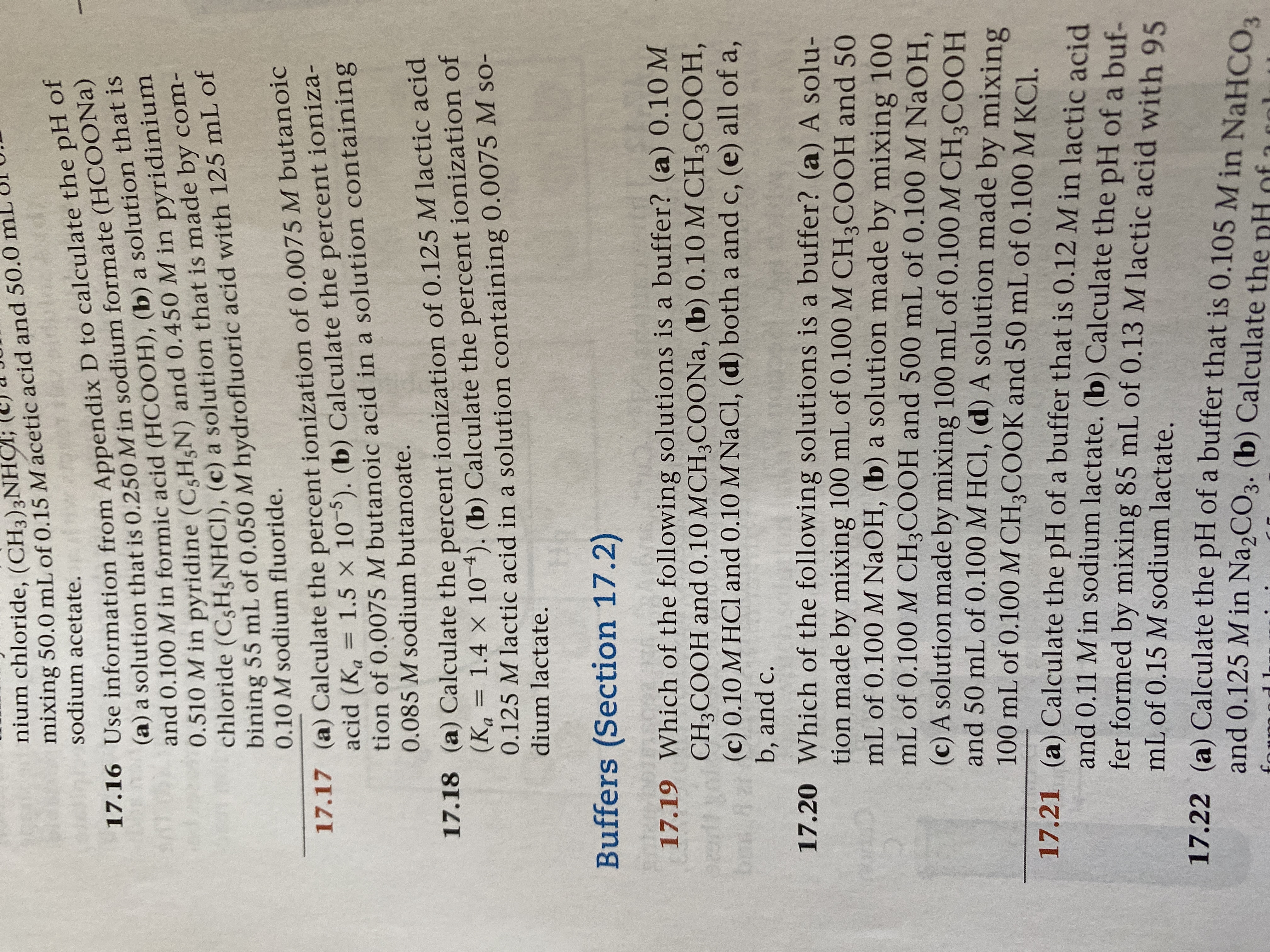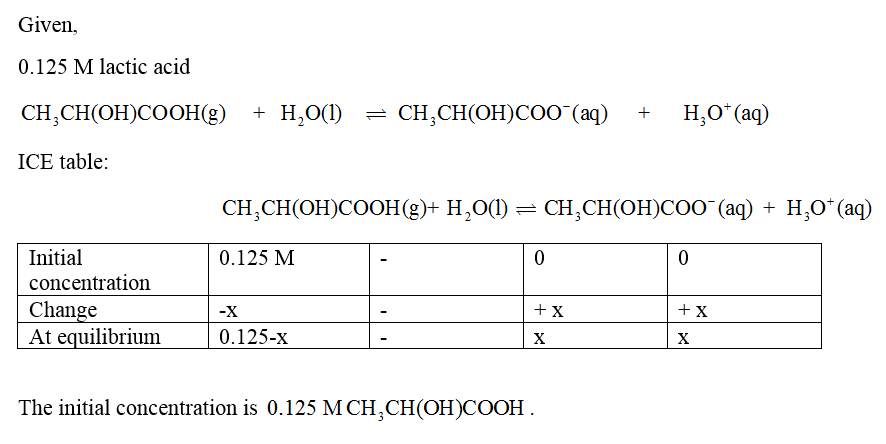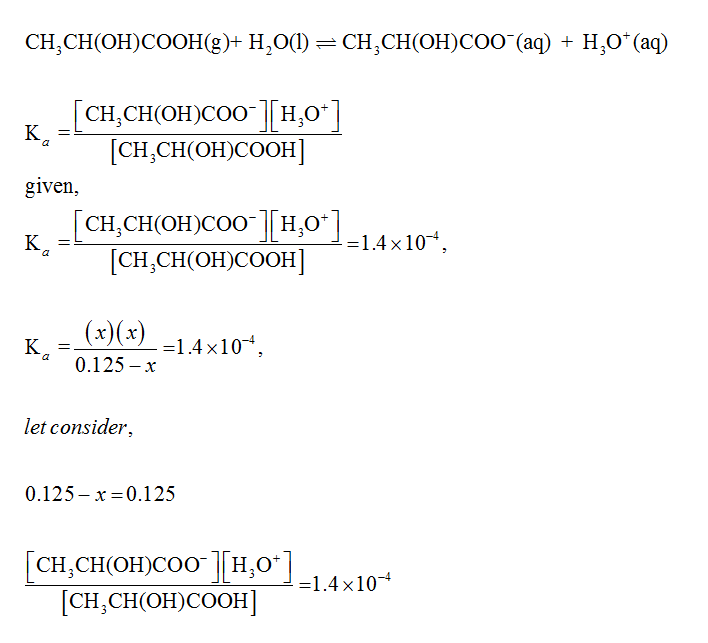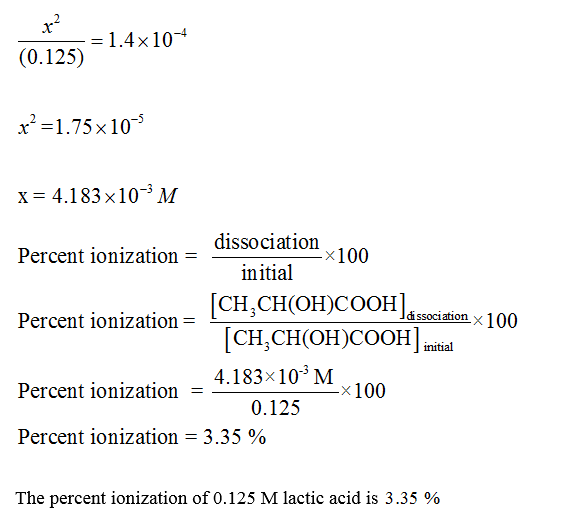# nium chloride, (CH3)3NHO;mixing 50.0 mL of 0.15 M acetic acid and 50.0sodium acetate.otocAd)(a) a solution that is 0.250M in sodium formate (HOCOONA)and 0.100 M in formic acid (HCOOH), (b) a solution that is0.510 M in pyridine (C,H;N) and 0.450 M in pyridiniumchloride (C5H5NHCI), (c) a solution that is made by com-bining 55 mL of 0.050 M hydrofluoric acid with 125 mL of0.10 M sodium fluoride.17.16 Use information from Appendix D to calculate the pH ofacid (K, = 1.5 × 10-5). (b) Calculate the percent ioniza-tion of 0.0075 M butanoic acid in a solution containing0.085 M sodium butanoate.17.17 (a) Calculate the percent ionization of 0.0075 M butanoic17.18 (a) Calculate the percent ionization of 0.125 M lactic acid(Ka = 1.4 × 104). (b) Calculate the percent ionization of0.125 M lactic acid in a solution containing 0.0075 M so-dium lactate.Buffers (Section 17.2)17.19 Which of the following solutions is a buffer? (a) 0.10 MCH3COOH and 0.10 MCH3COONA, (b) 0.10M CH3COOH,(c) 0.10 M HCI and 0.10 M NaCl, (d) both a and c, (e) all of a,b, and c.17.20 Which of the following solutions is a buffer? (a) A solu-tion made by mixing 100 mL of 0.100 M CH;COOH and 50mL of 0.100 M NaOH, (b) a solution made by mixing 100mL of 0.100 M CH3COOH and 500 mL of 0.100 M NaOH,(c) A solution made by mixing 100 mL of 0.100 M CH3COOHand 50 mL of 0.100 M HCI, (d) A solution made by mixing100 mL of 0.100 M CH3COOK and 50 mL of 0.100 M KCI.17.21 (a) Calculate the pH of a buffer that is 0.12 M in lactic acidand 0.11 M in sodium lactate. (b) Calculate the pH of a buf-fer formed by mixing 85 mL of 0.13 M lactic acid with 95mL of 0.15 M sodium lactate.17.22 (a) Calculate the pH of a buffer that is 0.105 M in NaHCO,and 0.125 M in Na2CO3. (b) Calculate the pH of a g

Question
38 views

17.18help_outlineImage Transcriptionclosenium chloride, (CH3)3NHO; mixing 50.0 mL of 0.15 M acetic acid and 50.0 sodium acetate. otocAd) (a) a solution that is 0.250M in sodium formate (HOCOONA) and 0.100 M in formic acid (HCOOH), (b) a solution that is 0.510 M in pyridine (C,H;N) and 0.450 M in pyridinium chloride (C5H5NHCI), (c) a solution that is made by com- bining 55 mL of 0.050 M hydrofluoric acid with 125 mL of 0.10 M sodium fluoride. 17.16 Use information from Appendix D to calculate the pH of acid (K, = 1.5 × 10-5). (b) Calculate the percent ioniza- tion of 0.0075 M butanoic acid in a solution containing 0.085 M sodium butanoate. 17.17 (a) Calculate the percent ionization of 0.0075 M butanoic 17.18 (a) Calculate the percent ionization of 0.125 M lactic acid (Ka = 1.4 × 104). (b) Calculate the percent ionization of 0.125 M lactic acid in a solution containing 0.0075 M so- dium lactate. Buffers (Section 17.2) 17.19 Which of the following solutions is a buffer? (a) 0.10 M CH3COOH and 0.10 MCH3COONA, (b) 0.10M CH3COOH, (c) 0.10 M HCI and 0.10 M NaCl, (d) both a and c, (e) all of a, b, and c. 17.20 Which of the following solutions is a buffer? (a) A solu- tion made by mixing 100 mL of 0.100 M CH;COOH and 50 mL of 0.100 M NaOH, (b) a solution made by mixing 100 mL of 0.100 M CH3COOH and 500 mL of 0.100 M NaOH, (c) A solution made by mixing 100 mL of 0.100 M CH3COOH and 50 mL of 0.100 M HCI, (d) A solution made by mixing 100 mL of 0.100 M CH3COOK and 50 mL of 0.100 M KCI. 17.21 (a) Calculate the pH of a buffer that is 0.12 M in lactic acid and 0.11 M in sodium lactate. (b) Calculate the pH of a buf- fer formed by mixing 85 mL of 0.13 M lactic acid with 95 mL of 0.15 M sodium lactate. 17.22 (a) Calculate the pH of a buffer that is 0.105 M in NaHCO, and 0.125 M in Na2CO3. (b) Calculate the pH of a g fullscreen
check_circle

The percent ionization of 0.125 M lactic acid has to be calculated.Step 2Step 3...

### Want to see the full answer?

See Solution

#### Want to see this answer and more?

Solutions are written by subject experts who are available 24/7. Questions are typically answered within 1 hour.*

See Solution
*Response times may vary by subject and question.
Tagged in